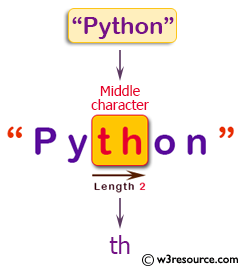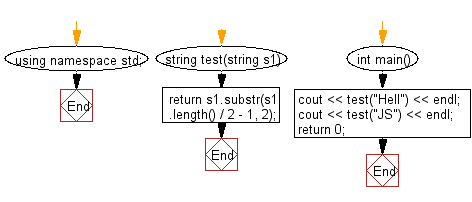﻿ C++ : New string of even length using the middle 2 character# C++ Exercises: Create a new string using the two middle characters of a given string of even length

## C++ Basic Algorithm: Exercise-67 with Solution

Write a C++ program to create a new string using the two middle characters of a given string of even length (at least 2).

Sample Solution:

C++ Code :

``````#include <iostream>
using namespace std;

string test(string s1)
{
return s1.substr(s1.length() / 2 - 1, 2);
}

int main()
{
cout << test("Hell") << endl;
cout << test("JS") << endl;
return 0;
}
``````

Sample Output:

```el
JS
```

Pictorial Presentation:Flowchart:C++ Code Editor: